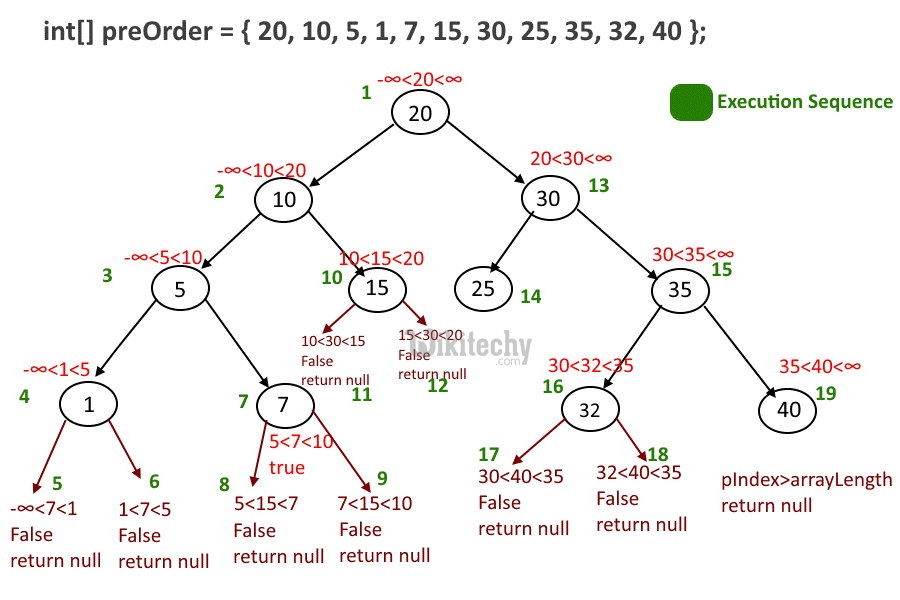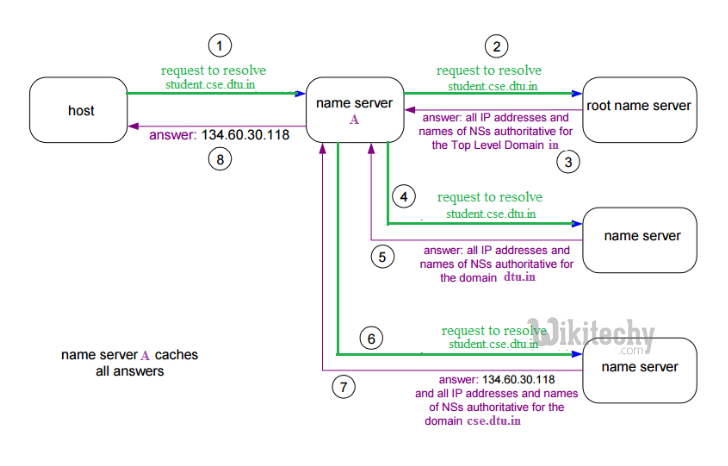# python tutorial - Python exam questions and answers - learn python - python programming

## How do you to implement the decorator function, using dollar()?

Here is the code:

## Output:Learn python - python tutorial - python-pre-order - python examples - python programs

## How to Convert domain to ip?

• If you have a file that lists domain names. you want to write a Python function that takes the file and returns a dictionary that has a key for the domain and a value for ip.
• For example, the input file ('domains.txt') looks like this:

## Output :Learn python - python tutorial - python-name-server - python examples - python programs

## How to count the number of instances?

• If you have a class A, and You want to count the number of A instances.
• Hint : use staticmethod

## How to do floor operation on using integers?

• To Write a floor division function without using '/' or '%' operator. For example, f(5,2) = 5/2 = 2, f(-5,2) = -5/2 = -3.

## How do you fetch every other item in the list?

• Suppose If you have a list like this:

We can use enumerate(L) to get indexed series:

This one may be the simplest solution:

## python interview questions :89

Will give the missing integer.

## How to Remove duplicate element from a list ?

Sometimes we may want to remove duplicate elements from a list:

This will print out:

Or simply we can do it: